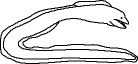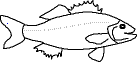EnchantedLearning.com is a user-supported site.
As a bonus, site members have access to a banner-ad-free version of the site, with print-friendly pages.

 You might also like: Divide Fruit into Four Groups Worksheet Printout Divide Ten Pets Worksheet Printout Divide Six by 2, 3, and 6 Worksheet Printout Divide Fruit into Three Groups Worksheet Printout Divide Fruit into Two Groups Worksheet Printout Today's featured page: Numbers in French: A Book to Print

 Go to the AnswersMath Pages EnchantedLearning.com Divide Fish by Five Divide the icy items into groups to do division. Visual Division PagesDivision Pages

 Divide the sharks into five equal groups.How many sharks are there? How many groups of sharks are there? How many sharks are in each group?
5 ÷ 5 =

 Divide the eels into five equal groups.How many eels are there? How many groups of eels are there? How many eels are in each group?
10 ÷ 5 =

 Divide the fish into five equal groups.How many fish are there? How many groups of fish are there? How many fish are in each group?
15 ÷ 5 =

## Enchanted Learning Search

 Search the Enchanted Learning website for: